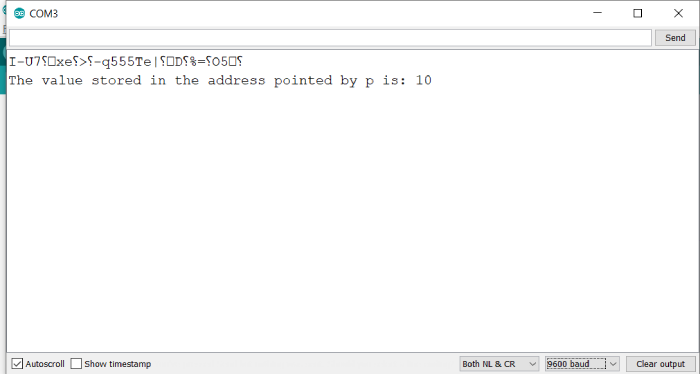# Reference and dereference operator in Arduino

The reference (&) and dereference operators (*) in Arduino are similar to C. Referencing and dereferencing are used with pointers.

• If x is a variable, then its address is represented by &x.

• Similarly, if p is a pointer, then the value contained in the address pointed to by p is represented by &p.

## Example

void setup() {
// put your setup code here, to run once:
Serial.begin(9600);
Serial.println();
int x = 10;
int *p;
p = &x; //p now contains the address of x
Serial.print("The value stored in the address pointed by p is: ");Serial.println(*p);
}

void loop() {
// put your main code here, to run repeatedly:
}

## Output

The Serial Monitor output is −